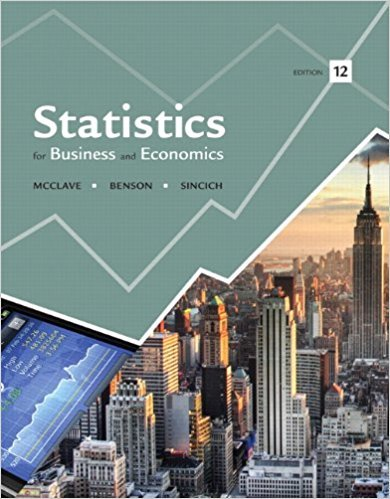×
×

# In each case, find the approximate sample size required toISBN: 9780321826237 51

## Solution for problem 62E Chapter 6

Statistics for Business and Economics | 12th Edition

• Textbook Solutions
• 2901 Step-by-step solutions solved by professors and subject experts
• Get 24/7 help from StudySoup virtual teaching assistantsStatistics for Business and Economics | 12th Edition

4 5 0 404 Reviews
15
2
Problem 62E

In each case, find the approximate sample size required to construct a 95% confidence interval for p that has margin of error of ME = .08.

a. Assume p is near .2.

b. Assume you have no prior knowledge about p, but you wish to be certain that your sample is large enough to achieve the specified accuracy for the estimate.

Step-by-Step Solution:

Step 1 of 2:

Now we have to find the approximate sample size required to a 95% confidence interval for p that has of margin of error of ME = 0.08.

Our goal is :

a). We assume that p is near 0.2.

b). We assume that you have no prior knowledge about p, but you wish to be certain that your sample is large enough to achieve the specified accuracy for the same.

a). We assume that p is near 0.2.

Now we have to compute sample size n.

The formula for n is

n =We know that level of significanceThen,Using the normal distribution tables,We know that p=0.2 and

q = 1-p

q = 1-0.2

q = 0.8

Therefore, q=0.8.

Then given ME = 0.08.

n =n =n =n = 96.0497

Hence we would need to take a sample of size is 97.

Step 2 of 2

##### ISBN: 9780321826237

Statistics for Business and Economics was written by and is associated to the ISBN: 9780321826237. The full step-by-step solution to problem: 62E from chapter: 6 was answered by , our top Business solution expert on 07/21/17, 05:42AM. This textbook survival guide was created for the textbook: Statistics for Business and Economics , edition: 12. Since the solution to 62E from 6 chapter was answered, more than 336 students have viewed the full step-by-step answer. The answer to “In each case, find the approximate sample size required to construct a 95% confidence interval for p that has margin of error of ME = .08.a. Assume p is near .2.b. Assume you have no prior knowledge about p, but you wish to be certain that your sample is large enough to achieve the specified accuracy for the estimate.” is broken down into a number of easy to follow steps, and 59 words. This full solution covers the following key subjects: sample, interval, approximate, assume, Case. This expansive textbook survival guide covers 15 chapters, and 1630 solutions.

Unlock Textbook Solution